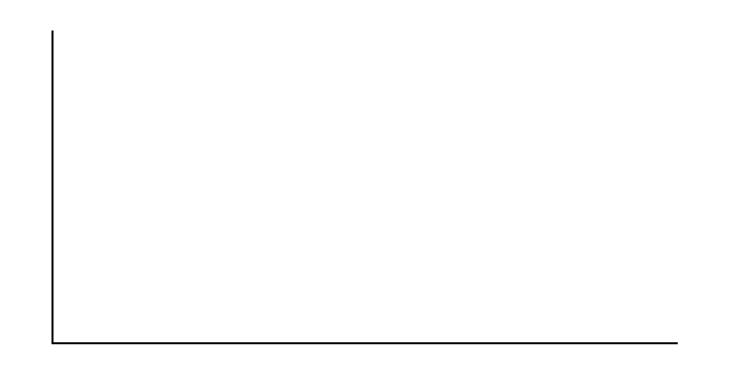Linear Regression and Correlation

# Regression (Fuel Efficiency)

Regression (Fuel Efficiency)

Class Time:

Names:

Student Learning Outcomes
• The student will calculate and construct the line of best fit between two variables.
• The student will evaluate the relationship between two variables to determine if that relationship is significant.

Collect the DataFind a reputable source that provides information on total fuel efficiency (in miles per gallon) and weight (in pounds) of new model cars with automatic transmissions. We will use this data to determine the relationship, if any, between the fuel efficiency of a car and its weight.

1. Using your random number generator, randomly select 20 cars from the list and record their weights and fuel efficiency into (Figure).
Weight Fuel Efficiency
2. Which variable should be the dependent variable and which should be the independent variable? Why?
3. By hand, do a scatterplot of “weight” vs. “fuel efficiency”. Plot the points on graph paper. Label both axes with words. Scale both axes accurately.Analyze the Data Enter your data into your calculator or computer. Write the linear equation, rounding to 4 decimal places.

1. Calculate the following:
1. a = ______
2. b = ______
3. correlation = ______
4. n = ______
5. equation: ŷ = ______
2. Obtain the graph of the regression line on your calculator. Sketch the regression line on the same axes as your scatter plot.
Discussion Questions
1. Is the correlation significant? Explain how you determined this in complete sentences.
2. Is the relationship a positive one or a negative one? Explain how you can tell and what this means in terms of weight and fuel efficiency.
3. In one or two complete sentences, what is the practical interpretation of the slope of the least squares line in terms of fuel efficiency and weight?
4. For a car that weighs 4,000 pounds, predict its fuel efficiency. Include units.
5. Can we predict the fuel efficiency of a car that weighs 10,000 pounds using the least squares line? Explain why or why not.
6. Answer each question in complete sentences.
1. Does the line seem to fit the data? Why or why not?
2. What does the correlation imply about the relationship between fuel efficiency and weight of a car? Is this what you expected?
7. Are there any outliers? If so, which point is an outlier?